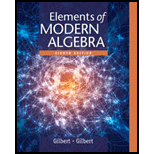# In Example 3 of Section 3.1, find elements a and b of S ( A ) such that ( a b ) − 1 ≠ a − 1 b − 1 . From Example 3 of section 3.1: A = { 1 , 2 , 3 } and S ( A ) is a set of bijective functions defined on A .### Elements Of Modern Algebra

8th Edition
Gilbert + 2 others
Publisher: Cengage Learning,
ISBN: 9781285463230

#### Solutions

Chapter
Section### Elements Of Modern Algebra

8th Edition
Gilbert + 2 others
Publisher: Cengage Learning,
ISBN: 9781285463230
Chapter 3.2, Problem 5E
Textbook Problem
1 views

## In Example 3 of Section 3.1, find elements a and b of S ( A ) such that ( a b ) − 1 ≠ a − 1 b − 1 .From Example 3 of section 3.1: A = { 1 , 2 , 3 } and S ( A ) is a set of bijective functions defined on A .

To determine

The elements a and b of S(A) such that (ab)1a1b1

### Explanation of Solution

Given information:

A={1,2,3} and S(A) set of functions defined from A to A.

Formula used:

1) Composition of function: Let f and g be the functions defined from A to A, then fg:AA, is defined as fg(x)=f[g(x)]

2) Definition of a group.

Suppose the binary operation is defined for element of a set G. The set G is a group with respect to , provided the following conditions hold.

1. G is closed under . That is xG and yG imply that xy is in G.

2. is associative. For all x,y,z in G, x(yz)=(xy)z.

3. G has an identity element e. There is an e in G such that xe=ex=x for all xG.

4. G contains inverses. For each aG, there exists bG such that ab=ba=e.

Calculation:

Let A={1,2,3}, S(A) be a set of bijective functions defined on A.

f(1) have three choices, f(2) have two choices and f(3) has only one choice.

So there are 3!=321=6 different mappings given by,

e={e(1)=1e(2)=2e(3)=3, σ={σ(1)=2σ(2)=1σ(3)=3, ρ={ρ(1)=2ρ(2)=3ρ(3)=1

γ={γ(1)=3γ(2)=2γ(3)=1, τ={τ(1)=3τ(2)=1τ(3)=2, δ={δ(1)=1δ(2)=3δ(3)=2

Thus, S(A)={e,ρ,τ,σ,γ,δ}.

In constructing the table for S(A), list the elements of S(A) in a column at the left

and in a row at the top.

Let e as an identity map, therefore

eρ=ρ,eτ=τ,eσ=σ,eγ=γ,eδ=δ

Similarly,

ρe=ρ,τe=τ,σe=σ,γe=γ,δe=δ.

When the product ρ2=ρρ is computed, then

ρ2(1)=ρ[ρ(1)]=ρ(2)=3ρ2(2)=ρ[ρ(2)]=ρ(3)=1ρ2(3)=ρ[ρ(3)]=ρ(1)=2ρρ=τ

Similarly, ρσ=γ, σρ=δ and so on.

Therefore, Cayley’s table for S(A) is given by,

eρτσγδeeρτσγδρρτeγδσττeρδσγσσδγeτργγσδρeτδδγστρe

Composition of e with other elements of S(A) satisfy the property,

(ab)1=a1b1

From the Cayley’s table,

a.

(ρρ)1=τ1=ρ,ρ1ρ1=ττ=ρ(ρρ)1=ρ1ρ1

b.

(ρτ)1=e1=e,ρ1τ1=τρ=e(ρτ)1=ρ1τ1

c.

(ρσ)1=γ1=γ,ρ1σ1=τσ=δ(ρσ)1ρ1σ1

d.

(ργ)1=δ1=δ,ρ1γ1=τγ=σ(ργ)1ρ1γ1

e.

(ρδ)1=σ1=σ,ρ1δ1=τδ=γ(ρδ)1ρ1δ1

f.

(τρ)1=e1=e,τ1ρ1=ρτ=e(τρ)1=τ1ρ1

g.

(ττ)1=ρ1=τ,τ1τ1=ρρ=τ(ττ)1=τ1τ1

h.

(τσ)1=δ1=δ,τ1σ1=ρσ=γ(τσ)1τ1σ1

i

### Still sussing out bartleby?

Check out a sample textbook solution.

See a sample solution

#### The Solution to Your Study Problems

Bartleby provides explanations to thousands of textbook problems written by our experts, many with advanced degrees!

Get Started

Find more solutions based on key concepts

Elementary Technical Mathematics

Mobile DEVICE Usage The average time U.S. adults spent per day on mobile devices (in minutes) for the years 200...

Applied Calculus for the Managerial, Life, and Social Sciences: A Brief Approach

In Problems 1-8, find the derivative of each function. 8.

Mathematical Applications for the Management, Life, and Social Sciences

Finding a Limit In Exercises 5-18, find the limit. limx3(x2+3x)

Calculus: Early Transcendental Functions (MindTap Course List)

For a given M 0, the corresponding in the definition of limx012x4= is: a) 2M4 b) 2M4 c) 12M4 d) 12M4

Study Guide for Stewart's Single Variable Calculus: Early Transcendentals, 8th